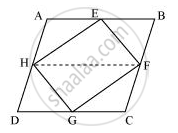# If E, F, G and H are respectively the mid-points of the sides of a parallelogram ABCD show that ar (EFGH) = 1/2ar (ABCD) - Mathematics

If E, F, G and H are respectively the mid-points of the sides of a parallelogram ABCD show that ar (EFGH) = 1/2ar (ABCD)

#### SolutionLet us join HF.

In parallelogram ABCD,

AD = BC and AD || BC (Opposite sides of a parallelogram are equal and parallel)

AB = CD (Opposite sides of a parallelogram are equal)

⇒ 1/2AD = 1/2BC and AH || BF

⇒ AH = BF and AH || BF (∵ H and F are the mid-points of AD and BC)

Therefore, ABFH is a parallelogram.

Since ΔHEF and parallelogram ABFH are on the same base HF and between the same parallel lines AB and HF,

∴ Area (ΔHEF) = 1/2Area (ABFH) ... (1)

Similarly, it can be proved that

Area (ΔHGF) = 1/2Area (HDCF) ... (2)

On adding equations (1) and (2), we obtain

Area(ΔHEF) + Area(ΔHGF) = 1/2Area (ABFH) + 1/2Area (HDCF)

= 1/2[Area (ABFH) + Area (HDCF)]

⇒ Area(EFGH) = 1/2Area(ABCD)

Concept: Theorem: Parallelograms on the Same Base and Between the Same Parallels.
Is there an error in this question or solution?
Chapter 9: Areas of Parallelograms and Triangles - Exercise 9.2 [Page 159]

#### APPEARS IN

NCERT Class 9 Maths
Chapter 9 Areas of Parallelograms and Triangles
Exercise 9.2 | Q 2 | Page 159

Share